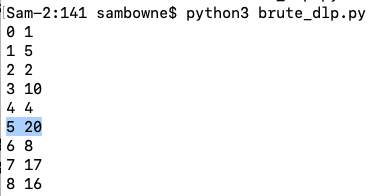# C 506: Baby-Step, Giant-Step Attack on DLP (50 pts)

What you need:
• Any computer with Python 3.

## Purpose

To crack the Discrete Logarithm Problem for small key sizes.

## Problem Statement

• On a ring with a prime modulus p
• And a base integer g
• We know an integer x
• We want to find an integer y such that:
gy = x

## Brute Force Attack

Let's use these values:
• p = 23
• g = 5
• x = 20
Create a file named brute_dlp.py containing this code:
```p = 23 g = 5 for i in range(p): print(i, pow(g, i, p)) ```
Run this program. As shown below, the answer is 5.## C 506.1: Brute Force (5 pts)

Find y from these values:
• p = 13337
• g = 13
• x = 1337
There are two solutions. The lower one is the flag.

## Larger p

What about a larger p, such as 5915587277?

Try running the program and you will see that it can quickly calculate 1 million values, but it will take a long time to try that many.

## Baby-Step Giant-Step Theory

We know this:
g y = x
We are looking for y, which could be from 1 to p - 1.

We break x into two pieces using m, which is the square root of p, rounded up to the nearest integer:

y = im + j
Where i and j are both between 0 and m. That is, we take i giant steps and j baby steps.

Therefore, we have:

g im + j = x
g j = x (g -m) i
We make a table of all possible g j values. Then we pick a value for m. Then we try values for j until a match is found in the table of g j values.

This is a time-memory trade-off algorithm.

## Algorithm

1. Calculate m = Ceiling(sqrt(p))

2. Calculate all the baby steps g j for j from 0 to m and save them in a table

3. Compute g -m

4. Loop through i from 0 to m

5. Look in the table for x (g -m) i. If it's found, y is im + j

6. If it's not found, increment i and go to step 5.

## Calculating g -m

Fermat's little theorem states, for any integer a:
a p = a (mod p)
or
a (p - 1) = 1 (mod p)
So, setting a to g m:
(g m) (p - 1) = g m * (p - 1) = 1 (mod p)
Multiplying both sides by g -m:
g m * (p - 2) = g -m (mod p)

## C 506.2: Find m (5 pts)

Find m for this value:
• p = 5915587277
The value of m is the flag.

Hint: use the Decimal library.

## C 506.3: Find g -m (5 pts)

For these values:
• p = 5915587277
• g = 497
Calculate g -m. That's the flag. The value of j is the flag.

## C 506.4: Baby Steps (10 pts)

For these values:
• p = 5915587277
• g = 497
Create the Baby Steps table. Find the j such that:
g j = 3457701878 (mod p)
The value of j is the flag.

## C 506.5: Crack DLP (15 pts)

Put all the pieces together and find y for these values:
• p = 5915587277
• g = 497
• x = 5751318998
The value of y is the flag.

Hint: use a dictionary

## C 506.6: Crack DLP (10 pts)

Put all the pieces together and find y for these values:
• p = 777737777777777
• g = 7777
• x = 719517368978140
The value of y is the flag.

This took about 3 minutes on my system.

Posted 9-6-20 by Sam Bowne
Point total corrected 11-11-21